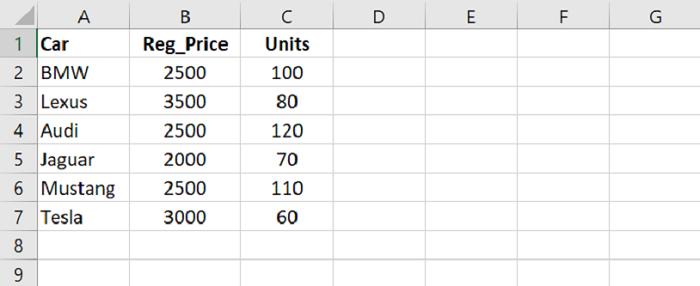# Python Pandas - Select a subset of rows and columns combined

To select a subset of rows and columns, use the loc. Use the index operator i.e. the square bracket and set conditions in the loc.

Let’s say the following are the contents of our CSV file opened in Microsoft Excel −At first, load data from a CSV file into a Pandas DataFrame −

dataFrame = pd.read_csv("C:\Users\amit_\Desktop\SalesData.csv")

Select a subset of rows and columns combined. Right column displays the column you want to display i.e. Cars column here −

dataFrame.loc[dataFrame["Units"] > 100, "Car"]

## Example

Following is the code −

import pandas as pd

# Load data from a CSV file into a Pandas DataFrame:

# selecting a subset of rows
print("\nSelect cars with Units more than 100: \n",dataFrame[dataFrame["Units"] > 100])

# displaying only two columns
res = dataFrame[['Reg_Price','Units']];
print("\nDisplaying only two columns : \n",res)

# Select a subset of rows and columns combined
# Right column displays the column you want to display i.e. Cars column here
res2 = dataFrame.loc[dataFrame["Units"] > 100, "Car"]

# display subset
print("\nSubset...\n",res2)

## Output

This will produce the following output −

Reading the CSV file...
Car   Reg_Price   Units
0      BMW        2500     100
1    Lexus        3500      80
2     Audi        2500     120
3   Jaguar        2000      70
4  Mustang        2500     110

Select cars with Units more than 100:
Car   Reg_Price   Units
2     Audi        2500     120
4  Mustang        2500     110

Displaying only two columns :
Reg_Price   Units
0        2500     100
1        3500      80
2        2500     120
3        2000      70
4        2500     110

Subset...
2 Audi
4 Mustang
Name: Car, dtype: object